# The acidity of ortho-nitrophenol is -----that of the ortho- methoxyphenol.(A) More than(B) Similar(C) Less than(D) None of theseVerified
178.2k+ views
Hint: Electron withdrawing group has $\text{-I}$(negative inductive effect) or negative mesomeric effect while electron donating group has $\text{+I}$(positive inductive effect) or positive mesomeric effect.
Acidic strength of a compound depends on the stability of the conjugate base.
In ortho-nitrophenol a nitro$\text{(-N}{{\text{O}}_{\text{2}}}\text{)}$group is attached to the ortho position (1, 2 –position) of phenol, while in ortho-methoxyphenol a methoxy$\text{(-OC}{{\text{H}}_{\text{3}}}\text{)}$group is attached to the ortho position (1, 2 –position) of phenol.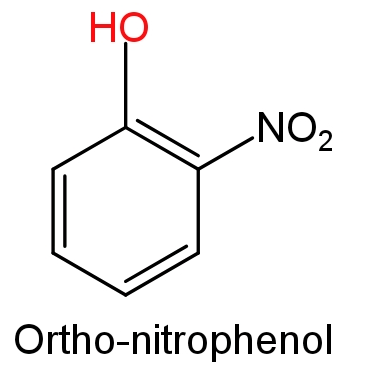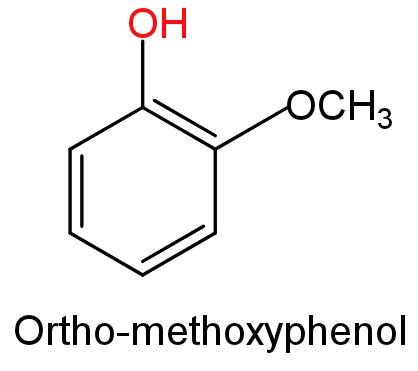The stability of phenoxide ion determines the acidic nature of the compounds.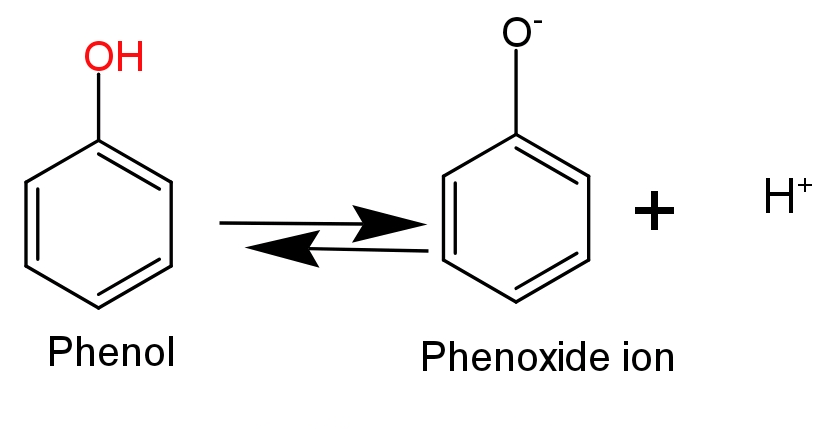-Ortho-nitrophenol is more acidic in nature because, in ortho-nitrophenol an electron withdrawing nitro$\text{(-N}{{\text{O}}_{\text{2}}}\text{)}$group is attached to the ortho position which helps in stabilising the negative charge on the oxygen atom of phenoxide ion. Hence, due to the stabilization of conjugate base of phenol acidic nature of phenol will increase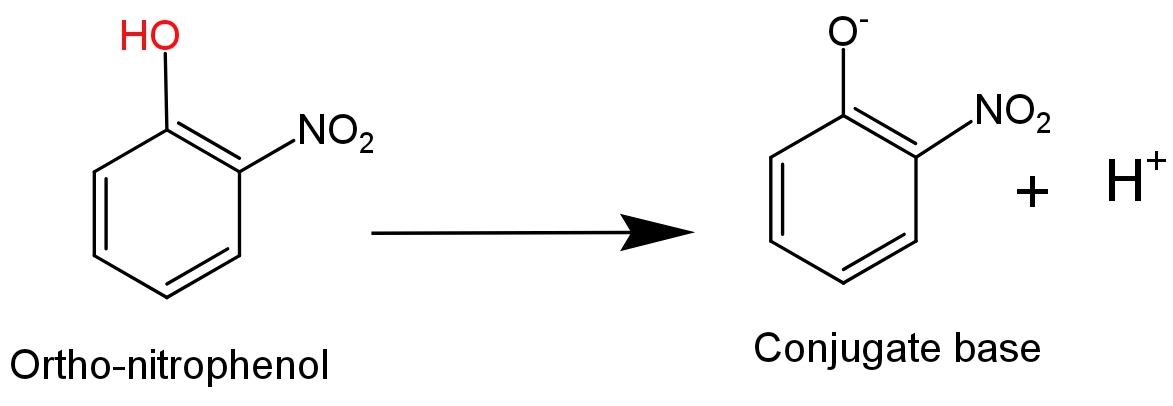- While in case of ortho-methoxyphenol an electron donating methoxy$\text{(-OC}{{\text{H}}_{\text{3}}}\text{)}$group is attached to the ortho position which destabilise the phenoxide ion by increasing the charge density on the oxygen atom of phenoxide ion. So, it will decrease the acidity of phenol.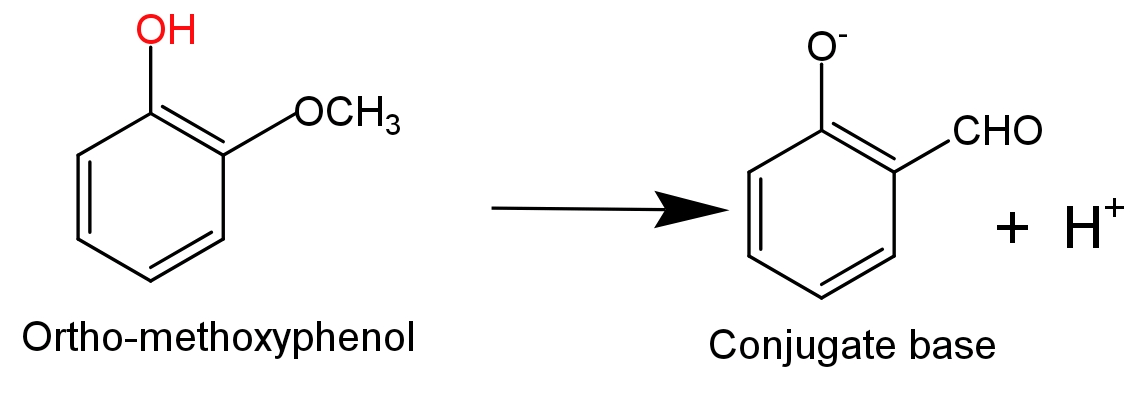Hence, option (A) will be the correct answer.

Note:
Inductive effect $\text{(I-effect)}$ is the permanent effect on sigma electrons. It involves the partial electron displacement along the chain of saturated carbon atoms due to the presence of covalent bonds at one end of the chain. While in mesomeric effect ($\text{(M-effect)}$involves the complete transfer of a pie- electron or lone pair of electrons to the adjacent atom or covalent bond.